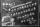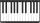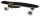# Fraction calculator

The calculator performs basic and advanced operations with fractions, expressions with fractions combined with integers, decimals, and mixed numbers. It also shows detailed step-by-step information about the fraction calculation procedure. Solve problems with two, three, or more fractions and numbers in one expression.

## Result:

### 1/3 + 1/5 = 8/15 ≅ 0.5333333

Spelled result in words is eight fifteenths.

### How do you solve fractions step by step?

1. Add: 1/3 + 1/5 = 1 · 5/3 · 5 + 1 · 3/5 · 3 = 5/15 + 3/15 = 5 + 3/15 = 8/15
For adding, subtracting, and comparing fractions, it is suitable to adjust both fractions to a common (equal, identical) denominator. The common denominator you can calculate as the least common multiple of both denominators - LCM(3, 5) = 15. In practice, it is enough to find the common denominator (not necessarily the lowest) by multiplying the denominators: 3 × 5 = 15. In the following intermediate step, the fraction result cannot be further simplified by canceling.
In other words - one third plus one fifth = eight fifteenths.

#### Rules for expressions with fractions:

Fractions - use the slash “/” between the numerator and denominator, i.e., for five-hundredths, enter 5/100. If you are using mixed numbers, be sure to leave a single space between the whole and fraction part.
The slash separates the numerator (number above a fraction line) and denominator (number below).

Mixed numerals (mixed fractions or mixed numbers) write as non-zero integer separated by one space and fraction i.e., 1 2/3 (having the same sign). An example of a negative mixed fraction: -5 1/2.
Because slash is both signs for fraction line and division, we recommended use colon (:) as the operator of division fractions i.e., 1/2 : 3.

Decimals (decimal numbers) enter with a decimal point . and they are automatically converted to fractions - i.e. 1.45.

The colon : and slash / is the symbol of division. Can be used to divide mixed numbers 1 2/3 : 4 3/8 or can be used for write complex fractions i.e. 1/2 : 1/3.
An asterisk * or × is the symbol for multiplication.
Plus + is addition, minus sign - is subtraction and ()[] is mathematical parentheses.
The exponentiation/power symbol is ^ - for example: (7/8-4/5)^2 = (7/8-4/5)2

#### Examples:

subtracting fractions: 2/3 - 1/2
multiplying fractions: 7/8 * 3/9
dividing Fractions: 1/2 : 3/4
exponentiation of fraction: 3/5^3
fractional exponents: 16 ^ 1/2
adding fractions and mixed numbers: 8/5 + 6 2/7
dividing integer and fraction: 5 ÷ 1/2
complex fractions: 5/8 : 2 2/3
decimal to fraction: 0.625
Fraction to Decimal: 1/4
Fraction to Percent: 1/8 %
comparing fractions: 1/4 2/3
multiplying a fraction by a whole number: 6 * 3/4
square root of a fraction: sqrt(1/16)
reducing or simplifying the fraction (simplification) - dividing the numerator and denominator of a fraction by the same non-zero number - equivalent fraction: 4/22
expression with brackets: 1/3 * (1/2 - 3 3/8)
compound fraction: 3/4 of 5/7
fractions multiple: 2/3 of 3/5
divide to find the quotient: 3/5 ÷ 2/3

The calculator follows well-known rules for order of operations. The most common mnemonics for remembering this order of operations are:
PEMDAS - Parentheses, Exponents, Multiplication, Division, Addition, Subtraction.
BEDMAS - Brackets, Exponents, Division, Multiplication, Addition, Subtraction
BODMAS - Brackets, Of or Order, Division, Multiplication, Addition, Subtraction.
GEMDAS - Grouping Symbols - brackets (){}, Exponents, Multiplication, Division, Addition, Subtraction.
Be careful, always do multiplication and division before addition and subtraction. Some operators (+ and -) and (* and /) has the same priority and then must evaluate from left to right.

## Fractions in word problems:

• Denominator 2Denominator of a fraction is 5 and numerator is 7. Write the fraction .
• In the cafeteriaThere are 18 students in Jacob’s homeroom. Six students bring their lunch to school. The rest eat lunch in the cafeteria. In simplest form, what fraction of students eat lunch in the cafeteria?
• In fractionsAn ant climbs 2/5 of the pole on the first hour and climbs 1/4 of the pole on the next hour. What part of the pole does the ant climb in two hours?
• Fraction to decimalWrite the fraction 3/22 as a decimal.Max is working out 2/3+7/9. He says the answer is 9/12. What mistake have Max made?
• The recipeThe recipe they are following requires 7/8 cups of milk, Tom already put 3/8 cups of milk. How much milk should Lea add to follow the recipe?
• Scouts 44/7 of the students in a school are boys. If 3/8 of the boys are scouts, how many scouts are there in a school of 1878 students?
• In dividingIn dividing fractions, get the reciprocal of the divisor and change division symbol to multiplication symbol. 2/3 : 5/6
• ShopperEva spent 1/4 in one store and 1/3 in another. What fraction is left?
• Eq-fracSolve the following equation with fractions: h + 1/3 =5/3
• Larry 2Larry spends half of his workday teaching piano lessons. If he sees 6 students, each for the same amount of time, what fraction of his workday is spent with each student?
• A storeA store received a shipment of 240 skateboards. In three weeks, it sold 1/3 of those skateboards. How many skateboards did the store sell?
• Unknown numberI think the number - its sixth is 3 smaller than its third.